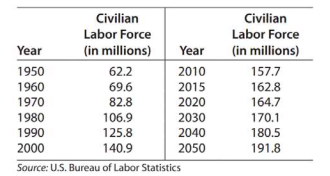# Modeling Civilian labor force The table gives the size of the U.S. civilian labor force (in millions) for selected years from 1950 and projected to 2050. Find a cubic function that models these data, with x equal to the number of years after 1950 and y equal to the labor force in millions. Report the model with three significant digit coefficients. Use the reported model to find the year when the second derivative predicts that the rate of change of the civilian labor force begins to decrease.### Mathematical Applications for the ...

11th Edition
Ronald J. Harshbarger + 1 other
Publisher: Cengage Learning
ISBN: 9781305108042

#### Solutions

Chapter
Section### Mathematical Applications for the ...

11th Edition
Ronald J. Harshbarger + 1 other
Publisher: Cengage Learning
ISBN: 9781305108042
Chapter 10.2, Problem 41E
Textbook Problem
1 views

## Modeling Civilian labor force The table gives the size of the U.S. civilian labor force (in millions) for selected years from 1950 and projected to 2050. Find a cubic function that models these data, with x equal to the number of years after 1950 and y equal to the labor force in millions. Report the model with three significant digit coefficients. Use the reported model to find the year when the second derivative predicts that the rate of change of the civilian labor force begins to decrease.This textbook solution is under construction.

### Still sussing out bartleby?

Check out a sample textbook solution.

See a sample solution

#### The Solution to Your Study Problems

Bartleby provides explanations to thousands of textbook problems written by our experts, many with advanced degrees!

Get Started